• 上机后的代码，内容为构建哈夫曼树，并求最短编码长度。
• 题目1172：哈夫曼树 时间限制：1 秒 内存限制：32 兆 特殊判题：否 题目描述： 哈夫曼树，第一行输入一个数n，表示叶结点的个数。需要用这些叶结点生成哈夫曼树，根据哈夫曼树的概念，这些结点有权值，即...
题目1172：哈夫曼树

时间限制：1 秒
内存限制：32 兆
特殊判题：否

题目描述：
哈夫曼树，第一行输入一个数n，表示叶结点的个数。需要用这些叶结点生成哈夫曼树，根据哈夫曼树的概念，这些结点有权值，即weight，题目需要输出所有结点的值与权值的乘积之和。
输入：
输入有多组数据。每组第一行输入一个数n，接着输入n个叶节点（叶节点权值不超过100，2<=n<=1000）。

输出：
输出权值。

样例输入：
5
1 2 2 5 9
样例输出：
37

#include <iostream>
#include<stdio.h>
#include<queue>
using namespace std;
priority_queue<int,vector<int>, greater<int> > Q;//建立一个小顶堆,注意最后的> >要分开写
int main()
{
int n;
while(scanf("%d",&n)!=EOF)
{
while(Q.empty()==false) Q.pop();//清空堆中元素
for(int i=0; i<n; i++)
{
int x;
scanf("%d",&x);
Q.push(x);//权值放入堆中
}
int ans=0;
while(Q.size()>1)
{
int a=Q.top();
Q.pop();
int b=Q.top();
Q.pop();
ans+=a+b;//父亲节点必为非叶子节点，故累加其权值
Q.push(a+b);//将双亲节点的权值放回堆中
}
printf("%d\n",ans);
}
return 0;
}

#include <iostream>
#include<stdio.h>
#include<queue>
#include<algorithm>
using namespace std;
int a;
bool  cmp(int a,int b)
{
return a<b;
}

int main()
{
int n;
while(scanf("%d",&n)!=EOF)
{
for(int i=0;i<n;i++)
{
scanf("%d",&a[i]);
}

int sum=0;
for(int i=0;i<n-1;i++)
{
sort(a,a+n,cmp);//每一次都要重新排序
a[i+1]+=a[i];
sum+=a[i+1];
}
printf("%d\n",sum);
}
return 0;
}

/**************************************************************
Problem: 1172
User: zhuoyuezai
Language: C++
Result: Accepted
Time:30 ms
Memory:1520 kb
****************************************************************/


展开全文• 给定一组具有确定权值的叶子节点，带权路径长度最小的二叉树就叫做哈夫曼树。 特点 1.权值越大的叶子节点越靠近根节点，而权值越小的叶子节点越远离根节点。 2.只有度为0和2的节点，不存在度为1的节点。 基本思想 ⑴...
定义
给定一组具有确定权值的叶子节点，带权路径长度最小的二叉树就叫做哈夫曼树。
特点
1.权值越大的叶子节点越靠近根节点，而权值越小的叶子节点越远离根节点。
2.只有度为0和2的节点，不存在度为1的节点。
基本思想
⑴ 初始化：由给定的n个权值{w1，w2，…，wn}构造n棵只有一个根结点的二叉树，从而得到一个二叉树集合F＝{T1，T2，…，Tn}；
⑵ 选取与合并：在F中选取根结点的权值最小的两棵二叉树分别作为左、右子树构造一棵新的二叉树，这棵新二叉树的根结点的权值为其左、右子树根结点的权值之和；
⑶ 删除与加入：在F中删除作为左、右子树的两棵二叉树，并将新建立的二叉树加入到F中；
⑷ 重复⑵、⑶两步，当集合F中只剩下一棵二叉树时，这棵二叉树便是哈夫曼树。
小提示
计算最短带权路径一定要理清思路，思路最关键，每个人的代码可能不一样，但思路是殊途同归的，有bug的话对学程序的人来说就见怪不怪了，具体在代码中体会，话不多说，直接上代码。
#include<bits/stdc++.h>
using namespace std;

typedef struct//哈夫曼树的存储表示
{
int weight;    					//权值
int parent, lChild, rChild;    	//双亲及左右孩子的下标
}HTNode, *HuffmanTree;

void Select(HuffmanTree hT, int n, int &s1, int &s2)//选择两个最小节点
{
s1 = s2 = 0;
int i;
for(i = 1; i < n; ++ i)//选择两个未有双亲的节点
{
if(hT[i].parent == 0)
{
if(0 == s1)
{
s1 = i;
}
else
{
s2 = i;
break;//后面一个for循环的标记
}
}
}
if(hT[s1].weight > hT[s2].weight)//确保s1>s2
{
int t = s1;
s1 = s2;
s2 = t;
}
for(i+=1;i<n;++i)//选择s2即break 故从i+1开始选择两个最小节点
{
if(hT[i].parent==0)
{
if(hT[i].weight < hT[s1].weight)
{
s2 = s1;
s1 = i;
}
else if(hT[i].weight < hT[s2].weight)
{
s2 = i;
}
}
}
//cout<<s1<<" "<<s2<<"**"<<endl;
}

void CreateHufmanTree(HuffmanTree &hT)//构造哈夫曼树
{
int n,m;
cin>>n;
m = 2*n - 1;
hT = new HTNode[m + 1];
hT.weight=m;  // 0号节点用来记录节点数量
for(int i = 1; i <= m; ++ i)
{
hT[i].parent = hT[i].lChild = hT[i].rChild = 0;//初始化
}
for(int i = 1; i <= n; ++ i)
{
cin >> hT[i].weight;    // 输入权值
}
for(int i = n + 1; i <= m; ++ i)//建立过程
{
int s1, s2;
Select(hT, i, s1, s2);
hT[s1].parent = hT[s2].parent = i;
hT[i].lChild = s1; hT[i].rChild = s2;    			//作为新节点的孩子
hT[i].weight = hT[s1].weight + hT[s2].weight;    	//新节点为左右孩子节点权值之和
}

}

int HuffmanTreeWPL_(HuffmanTree hT, int i, int deepth)//递归计算WPL
{
if(hT[i].lChild == 0 && hT[i].rChild == 0)
{
return hT[i].weight * deepth;
}
else
{
return HuffmanTreeWPL_(hT, hT[i].lChild, deepth + 1) + HuffmanTreeWPL_(hT, hT[i].rChild, deepth + 1);
}
}

int HuffmanTreeWPL(HuffmanTree hT)//计算WPL
{
return HuffmanTreeWPL_(hT, hT.weight, 0);
}

void DestoryHuffmanTree(HuffmanTree &hT)//销毁哈夫曼树
{
delete[] hT;
hT = NULL;
}

int main()
{
HuffmanTree hT;
CreateHufmanTree(hT);
cout << HuffmanTreeWPL(hT) << endl;
DestoryHuffmanTree(hT);
return 0;
}



展开全文• 自己写的哈夫曼树的构造和求最短路径，typedef struct { int weight; int parent; int lchild; int rchild; }HNodeType; int n;HNodeType HuffNode [MAXNODE]; void HaffmanTree(HNodeType HuffNode [ ])
• **描述：**哈夫曼编码是可变字长编码（VLC）的一种，该方法完全依据字符出现概率来构造异字头的平均长度最短的码字。 **贪心策略：**把编码映射成二叉树，把频率高的字符分配给靠近根节点的叶节点，把频率低的字符...
哈夫曼编码
描述：哈夫曼编码是可变字长编码（VLC）的一种，该方法完全依据字符出现概率来构造异字头的平均长度最短的码字。
贪心策略：把编码映射成二叉树，把频率高的字符分配给靠近根节点的叶节点，把频率低的字符放置在远离根节点的叶节点。
自底向上构造二叉编码树，由森林不断合并得到一棵二叉树。
思路分析：为了便于找到频次最低的字符，哈夫曼算法建立一个以f为键值的优先队列Q，假设编码字符集中每一字符c的频率是f( c ).哈夫曼编码算法以自底向上的方式构造最优编码树T。

一开始，每个字符构成只包含一个节点的树
合并频率最低的两棵树，并产生一棵新树，其频率为合并的两棵树的频率之和，并将新树插入优先队列Q中。
循环n-1次后，优先队列中只剩下一棵树，即最优二叉编码树。

struct cmp{
bool operator()(connst int &x,const int &y){
return x>y ;
}
};//定义有限对列比较函数
double haffmanCoding(int n,int *freq){
int i,total = 0,sumFreq = 0,jointFreq ;
priority_queue<int,vector<int>,cmp> heap ;
for(int i=0;i<n;i++){
total += freq[i] ;  //频率总和
heap.push(freq[i]) ;
}//形成有限对列
while(heap.size()>1){ //循环选择对列中频次最少的两个元素合并
jointFreq = 0 ;   //合并两个节点的频率
for(int i=0;i<2;i++){   //删除频次最少的两元素
jointFreq += heap.top() ;
heap.pop() ;
}
sumFreq += jointFreq ;
heap.push(jointFreq) ;   //优先队列中插入合并节点
}
return sumFreq/(1.0*total) ;   返回平均码长
}
//复杂度O(nlg(n))

单源最短路径
描述: 有向图G有n个顶点，给定每两个顶点的权值，权值为非负实数，如果权值为-1，表示没有边相连，默认第一个顶点为源。计算假设源可以到达任何一个顶点，从源到各顶点的最短路径长度。
特征：最短路径的子路径也是源到相应顶点的最短路径。
Dijstra算法：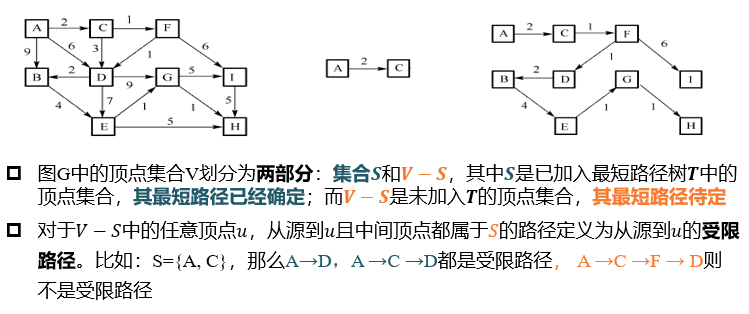贪心策略：选择集合V-S中受限路径长度最短的顶点，并把相应顶点加入到S中，相应地最短路径树T叶增加一条边。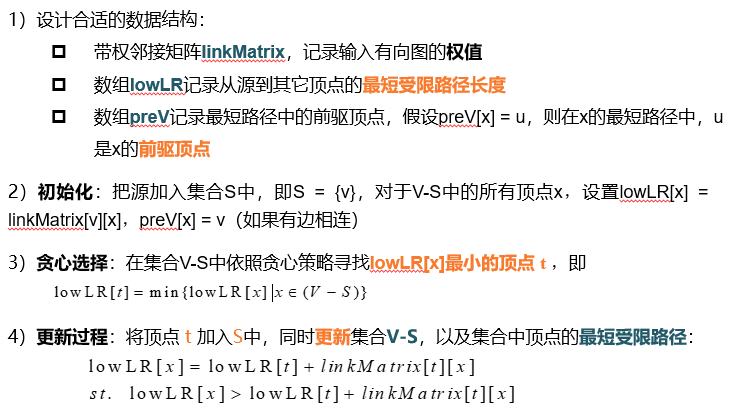代码：
#define INF 0x3f3f3f3f //预定义的充分大的数
#define MaxV 100
int preV[MaxV];  //最短路径树中的前驱节点信息表
int visited[MaxV];  //结点是否加入S的标记表，0是为加入，1是加入
void Dijkstra(int linkMatrix[][MaxV],int lowLR[MaxV],int numV,int beginV){
int i,j,min,newCost ;
memset(visited,0,sizeof(visited)) ;   //初始化，所有结点为加入
//开始结点beginV加入S
visited[beginV] = 1 ;
for(int i=0;i<numV;i++){
preV[i] = beginV ;
}
lowLR[beginV] = 0 ;
preV[beginV] = -1 ;  //树根的标记
int selectV = beginV ;
for(int i=1;i<numV;i++){
for(int j=0;j<numV;j++){
if(visited[j]==0&&newCost<lowLR[j]){
lowLR[j] = newCost ;
preV[j] = selectV ;
}
}
min = INF ;
for(int j=0;j<numVlj++){
if(visited[j]==0&&lowLR[j]<min){
min = lowLR[j] ;
selectV = j ;
}
}
visited[selectV] = 1 ;  //更新被选中顶点的状态
}
}

最小生成树MST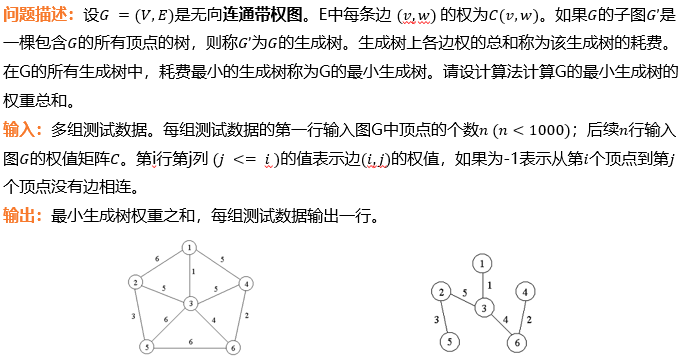最小生成树性质

生成树有V个顶点，V-1条边
生成树中增加一条边则会得到一个回路，回路中删除任何一条边又得到一棵树
设G=(V,E)是连通带权图，U是V的真子集。如果（u,v）属于E，其中u属于U，v属于（V-U），称之为跨边，且在所有这样的边中，(u,v)的权C(u,v)最小，那么一定存在G的一棵最小生成树，它包含边(u,v).
两种求解算法
Prim算法(加点法)：针对图中的顶点设计贪心策略，逐步添加点/边构造最小生成树
策略是选择最小跨边。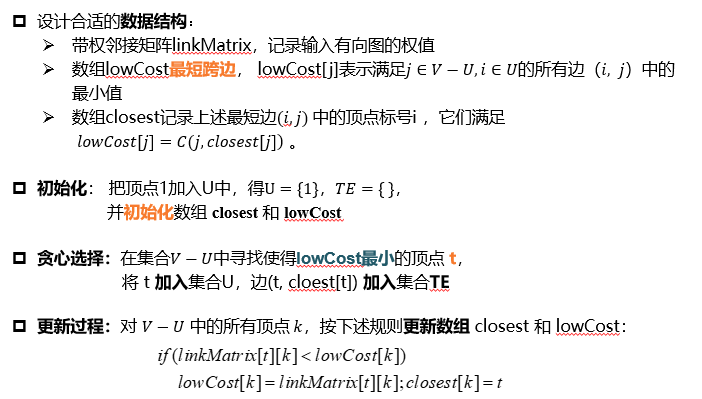代码：

#define MaxV 1000
#define INF 0x3f3f3f3f  //预定义充分大的值
int visited[MaxV] = {0} ;   //节点是否加入MIS的标记表，0表示未加入，1表示已加入
int lowCost[MaxV],closet[MaxV] ;
int i,k ;
int min,costMST = 0 ;
visited = 1 ;  //顶点加入MST
closetst = -1 ;  //树根的标记
for(int i=1;i<numV;i++){   //初始化
closest[i] = 0 ;
}
for(int i=0;i<numV;i++){
min = INF ;
int selectV = 0 ;
for(int k=1;k<numV;k++){    //贪心选择最短边
if(!visited[k]&&lowCost[k]<min){
min = lowCost[k] ;
selectV = k ;
}
}
costMST += min ;   //更新MST树权重
visited[selectV] = 1 ;
for(k=1;k<numV;k++){
closest[k] = selectV ;
}
}
}
return costMST ;
}
//O(|V|^2)

Kruskal算法（加边法）：针对图中的边设计贪心策略，逐步合并分支构造最小生成树，策略是选择最短边合并两个不连通分支。
这里先补充一下并查集的概念：并查集是一种树形的数据结构，常用于处理一些不想交集合的合并和查询问题，它包含两个基本操作：查询Find和合并Union.(每个集合找一个代表)，下面部分是讲解并查集的优化方法（采用了路径压缩），更详细的解释：https://blog.csdn.net/weixin_44508223/article/details/102648509
第一步Find:路径上的每个节点都直接连接在根上。递归的访问当前节点到树根的路径，改变每一个节点的引用到根节点。
//初始化father[]数组为-1，也就是，代表树中只有一个节点
int Find(int x){
int p = x ;
while(father[p]>0)
p = father[p] ;   //找到树根
return p ;
//将每一个节点都指向根节点
while(father[x]!=p&&father[x]>0){
int temp = father[x] ;
father[x] = p ;  //指向树根
x = temp ;
}
return p ;
}

第二步Union:按秩合并，即总是将更浅的树连接至更深的树上，避免增加合并数的秩。（这里的秩表示树的深度，单个元素的树的秩定义为0），当两个树的秩同为r时，它们的秩变为r+1,为了方便比较，把集合的秩的相反数存储在根节点。
void Union(int x,int y){
int xRoot = Find(x) ;
int yRoot = Find(y) ;
if(xRoot==yRoot)  return ;
if(-father[xRoot]>-father[yRoot])
father[yRoot] = xRoot ;
else{
if(father[xRoot] == father[yRoot])
father[yRoot] -= 1 ;   //相等
father[xRoot] = yRoot ;
}
}

Kruskal算法俗称闭环法，该算法的实现关键是在加边时避免出现环路，用并查集来实现连通分支查找和合并的相关操作。
一开始各个顶点是孤立的，然后选择边集中权值最小的边(i,j)，如果加入不产生回路，则加入，否则舍弃，直到加够n-1条边为止。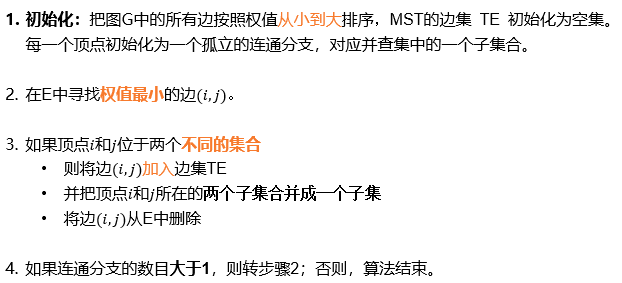int Kruskal(node juSet[MaxV],int numV,edge degeSet[MaxE],int numE){
qsort(edgeSet,numE,sizeof(struct edge),cmp) ;   //将边排序
int totalCost = 0,cntE = 0,fatherX,fatherY ;
for(int i=0;i<numE;i++){
fatherX = Find_Set(edgeSet[i].x) ;
fatherY = Find_Set(edgeSet[i].y) ;
if(fatherX!=fatherY){
Union(fatherX,fatherY) ;
totalCost += edgeSet[i].w ;
cntE++ ;
}
if(cntE == numV-1) // 完成
}
}
//O（elog(e)）



展开全文• （1）设需要编码的字符集为{d1, d2, …, dn}，它们出现的频率为{w1, w2, …, wn}，应用哈夫曼树构造最短的不等长编码方案。 提示： 哈夫曼树(Huffman Tree),又叫最优二叉树，指的是对于一组具有确定权值的叶子结点的...
内容：
（1）设需要编码的字符集为{d1, d2, …, dn}，它们出现的频率为{w1, w2, …, wn}，应用哈夫曼树构造最短的不等长编码方案。
提示：
哈夫曼树(Huffman Tree),又叫最优二叉树，指的是对于一组具有确定权值的叶子结点的具有最小带权路径长度的二叉树。
哈夫曼树的构造算法：假设有n个权值，则构造出得哈夫曼树有n个叶子结点。n个权值分别设为w1,w2,…,wn,则哈夫曼树的构造规则为:
(1)将w1,w2,…,wn看成是有n棵树的森林(每棵树仅有一个结点);
(2)在森林中选出两个根结点的权值最小的树合并，作为一棵新树的左、右子树，且新树的根结点权值为其左、右子树根结点权值之和；
(3)从森林中删除选取的两棵树，并将新树加入森林;
(4)重复(2)、(3)步，直到森林中只剩一棵树为止，该树即为所求得的哈夫曼树。
完整代码：
#include<iostream>
#include <stdlib.h>
using namespace std;
struct huffman
{
double weight;
int lchild,rchild,parent;
};

int Select(huffman huff[],int i)	//用来在数组huff中获得权值最小的根结点
{
double min=11000;
int min1;
for(int k=0;k<i;k++)
{
if(huff[k].weight<min&&huff[k].parent==-1)
{
min=huff[k].weight;
min1=k;
}
}
huff[min1].parent=1;
return min1;
}

void HuffmanTree(huffman huff[],double weight[],int n)	//建立哈夫曼树
{
for(int i=0;i<2*n-1;i++)	//初始化
{
huff[i].lchild=-1;
huff[i].parent=-1;
huff[i].rchild=-1;
}
for(int i=0;i<n;i++)	//构造n棵只含有根结点的二叉树
{
huff[i].weight=weight[i];
}
for(int k=n;k<2*n-1;k++)	//n-1次合并
{
int i1=Select(huff,k);	//得到当前权值最小的结点i1
int i2=Select(huff,k);	//得到当前权值最小的结点i2
huff[i1].parent=k;		//合并i1和i2两个结点为一棵新树的左右子树
huff[i2].parent=k;
huff[k].weight=	huff[i1].weight+huff[i2].weight;
huff[k].lchild=i2;
huff[k].rchild=i1;
}
}

void huffmancode(huffman huff[],int n)	//由哈夫曼树生成编码
{
string s;
int j;
for(int i=0;i<n;i++)
{
s="";
j=i;
while(huff[j].parent!=-1)	//当结点j不是根结点时循环
{
if(huff[huff[j].parent].lchild==j)	//当结点j是其父结点的左孩子时
s=s+"0";
else s=s+"1";
j=huff[j].parent;
}
cout<<"权值为"<<huff[i].weight<<"的霍夫曼编码为：";
for(int j=s.length()-1;j>=0;j--)	//将s逆序输出，得到结点i的编码
{
cout<<s[j];
}
cout<<endl;
}
}
int main()
{
huffman h;
int n;
cout<<"请输入权值的个数"<<endl;
cin>>n;
double *w = (double*)malloc(n*sizeof(double));
cout<<"请分别输入n个权值:\n"<<endl;
int i;
for(i=0; i<n; ++i)
{
cin>>w[i];
}
HuffmanTree(&h,w,n);
huffmancode(&h,n);
return 0;
}


运行结果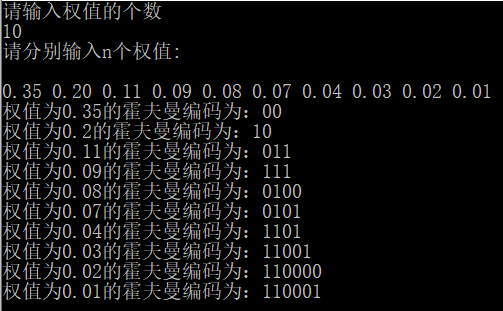展开全文• 哈夫曼树的带权路径长度是什么？1．树的路径长度树的路径长度是从树根到树中每一结点的路径长度之和。在结点数目相同的二叉树中，完全二叉树的路径长度最短。2．树的带权路径长度(Weighted Path Length of Tree，...
• #include #include #define n 7 //假设有七个节点元素 struct Element {  int flag;... //构建哈夫曼树  length=road(huffman,m);  printf("带权路径长度为:%d",length); }
• 哈夫曼树，又称最优二叉树，是一类带权路径长度最短的树。 构建哈夫曼树的算法如下： 对给定的n个权值{W1,W2,W3,...Wi,...,Wn}构成n个二叉树的初始集合F={T1,T2,T3,...Ti,...Tn},其中每棵二叉树Ti中只有一个权值为...
• 贪心思想和案例(活动安排问题,0-1背包问题,最优装载,哈夫曼编码,单源最短路径,最小生成(Prim,Kruskal),汽车加油问题)。算法课使用的ppt,可结合我的博客算法专栏一起看。有详细代码。
• 哈夫曼树：一类带权路径最短的树。用于通讯及数据传送中构造传输效率最高的二进制编码（哈夫曼编码），用于编程中构造平均执行时间最短的最佳判断过程。节点之间的路径长度：从一个节点到另一个节点之间的分支数目。...
• 哈夫曼树=带权路径长度最短的树（要么都比二叉树或者三叉树） 1.哈夫曼树权值越大的叶子越靠近根 2.具有相同带权节点的哈夫曼树不为一 步骤 1.构造森林全是根 2.选俩个小的为左右子树，根为权之和 3.将这棵树放进...
• 构造哈夫曼树：按照频率来分配的（频率递增是因为出现次数多，那么就越深权重越大），就是生成哈夫曼树，基于树形结构，然后再次插进队列里面。 频率低的，就断。频率高的就长 二、二路最佳合并 三、最小代价...算法 贪心算法
• 何为贪心  假设有四种硬币：二角五分、一角、五分和一分。现要找给顾客六角三分。  显然，会拿出2个二角五分、1个一角和3个一分的硬币交给顾客。  贪心方法思路：首先选出一个面值不超过六角三分的最大硬币，即二...贪心算法 c++
• 本篇博文将介绍什么是哈夫曼树，并且如何在java语言中构建一棵哈夫曼树，怎么利用哈夫曼树实现对文件的压缩和解压。...哈夫曼树是带权路径长度最短的树，权值较大的结点离根较近。(一)树的相关概念1.路径路径长...
• 树的带权路径长度(Weighted Path Length of ...哈夫曼树是一种带权路径长度最短的二叉树，也称为最优二叉树。   哈夫曼树构建教程 https://blog.csdn.net/xueba8/article/details/78477892 例：对于给定的一...
• ## 哈夫曼树结构和带权路径长度计算

万次阅读 多人点赞 2017-11-08 13:55:38
哈夫曼树是一种带权路径长度最短的二叉树，也称为最优二叉树。下面用一幅图来说明。 它们的带权路径长度分别为： 图a： WPL=5*2+7*2+2*2+13*2=54 图b： WPL=5*3+2*3+7*2+13*1=48 可见，...
• 本文转自哈夫曼树哈夫曼树编码 在一般的数据结构的书中，树的那章后面，著者一般都会介绍一下哈夫曼(HUFFMAN) 树和哈夫曼编码。哈夫曼编码是哈夫曼树的一个应用。哈夫曼编码应用广泛，如 JPEG中就应用...c++ 算法
• 哈夫曼树一、哈夫曼树的基本介绍1.1 什么是哈夫曼树1.2 哈夫曼树的重要概念1.3 哈夫曼树的创建思路二...哈夫曼树是带权路径长度最短的树，权值较大的节点离根较近。 1.2 哈夫曼树的重要概念 路径路径长度：在一棵树
• 博客中思维导图的高清PDF版本,可关注公众号 一起学计算机 点击 资源获取 获得 目录 8.2最优前缀码及哈夫曼算法 ​8.3哈夫曼算法的证明及应用 ​8.4最小生成 8.5Prim算法 ​8.6Kruskal算法 ​8.7单源最短路径问题......